## Directional derivative, definition and computation

Directional derivative, definition and computation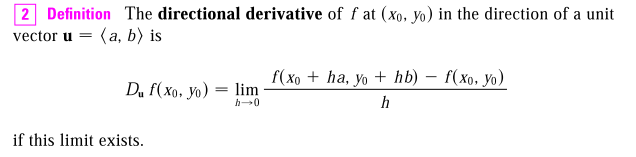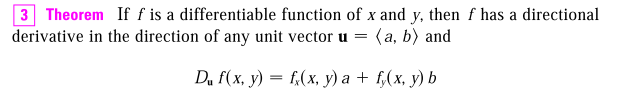The proof of this theorem is easy enough, just use the definition and do a small trick:

\begin{align*} f(x + ha, y + hb) - f(x, y) &= f(x + ha, y + hb) - f(x, y + hb) + f(x, y + hb) - f(x, y) \end{align*}

Directional derivative is also connected to gradient vector: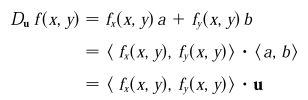In vector notation, directional derivative can be written as: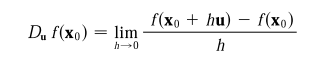or,

\begin{align*} D_uf(\mathbf{x}) &= \nabla f(\mathbf{x}) \cdot u \end{align*}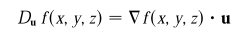Directional derivative can be maximized like this: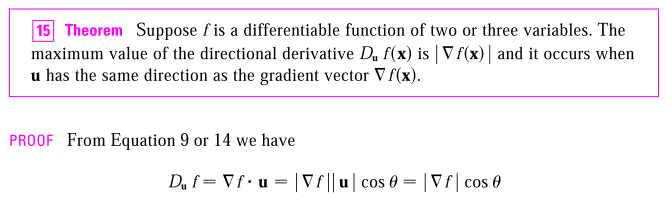As can be visualized in matlab: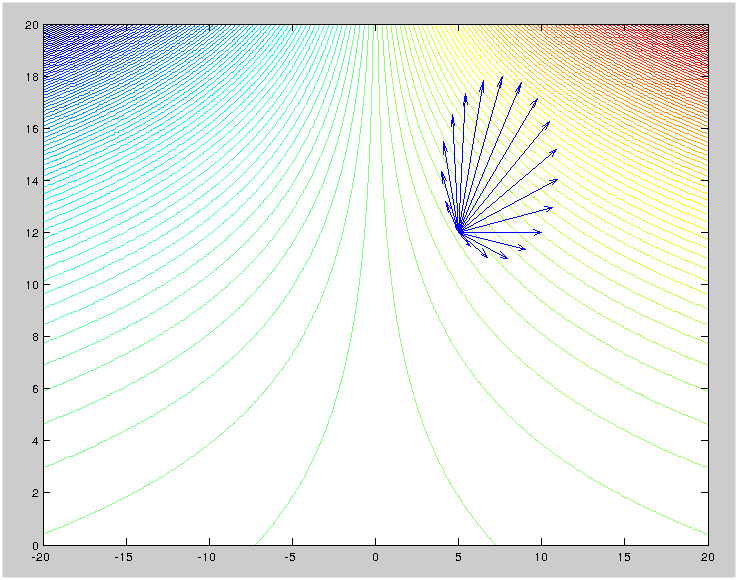Visualization of deepest gradient (matlab code)
Click to toggle code (may have to try twice)
x = linspace(-20,20, 300);
y = linspace(0,20,300);
[X,Y] = meshgrid(x,y);
a = .5
b = .2
Z = a .* X .* exp(b .* Y)
x0 = 5
y0 = 12
fx0 = a .* exp(b .* y0)
fy0 = a .* b .* x0 .* exp(b .* y0)
fxy = vertcat(fx0, fy0)
t = linspace(0, 2.*pi, 20)
cost = cos(t)'
sint = sin(t)'
tmat = horzcat(cost,  sint)
xvec = repmat(x0, size(uvec))
yvec = repmat(y0, size(uvec))
contour(X,Y,Z, 150)
hold on
quiver(xvec, yvec, uvec, vvec)


It's also related to (deepest) gradient descent algorithm: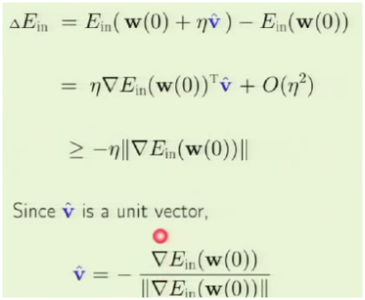Directional derivative is just a generalization of partial derivative. i.e. it need not be $\partial f / \partial x$, it need not be $\partial f / \partial x$, it could be something in between. The point need not move in the direction of x-axis, nor the y-axis, it could move in any direction. Maximization of directional derivative is finding the direction that results in fastest change.